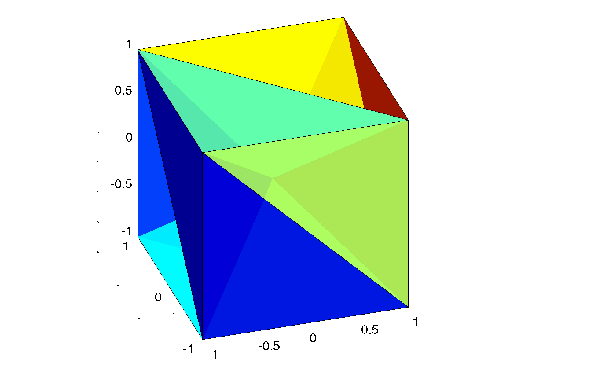MATLAB Function Referencedelaunay3

3-dimensional Delaunay tessellation

Syntax

• ```T = delaunay3(x,y,z)
```T = delaunay3(x,y,z,options)
``````

Description

```T = delaunay3(x,y,z) ``` returns an array `T`, each row of which contains the indices of the points in `(x,y,z)` that make up a tetrahedron in the tessellation of `(x,y,z)`. `T` is a `numtes`-by-`4` array where `numtes` is the number of facets in the tessellation. `x`, `y`, and `z` are vectors of equal length. If the original data points are collinear or `x`, `y`, and `z` define an insufficient number of points, the triangles cannot be computed and `delaunay3` returns an empty matrix.

`delaunay3` uses Qhull.

`T = delaunay3(x,y,z,options)` specifies a cell array of strings `options` to be used in Qhull via `delaunay3`. The default options are `{'Qt','Qbb','Qc'}`.

If `options` is `[]`, the default options are used. If `options` is `{''}`, no options are used, not even the default. For more information on Qhull and its options, see http://www.qhull.org.

Visualization

Use `tetramesh` to plot `delaunay3` output. `tetramesh` displays the tetrahedrons defined in `T` as mesh. `tetramesh` uses the default tranparency parameter value `'FaceAlpha' = 0.9`.

Examples

Example 1. This example generates a 3-dimensional Delaunay tessellation, then uses `tetramesh` to plot the tetrahedrons that form the corresponding simplex. `camorbit` rotates the camera position to provide a meaningful view of the figure.

• ```d = [-1 1];
[x,y,z] = meshgrid(d,d,d);  % A cube
x = [x(:);0];
y = [y(:);0];
z = [z(:);0];
% [x,y,z] are corners of a cube plus the center.
Tes = delaunay3(x,y,z)

Tes =

9   1   5   6
3   9   1   5
2   9   1   6
2   3   9   4
2   3   9   1
7   9   5   6
7   3   9   5
8   7   9   6
8   2   9   6
8   2   9   4
8   3   9   4
8   7   3   9

X = [x(:) y(:) z(:)];
tetramesh(Tes,X);camorbit(20,0)```

Example 2. The following example illustrates the `options` input for `delaunay3`.

• ```X = [-0.5 -0.5 -0.5 -0.5 0.5 0.5 0.5 0.5];
Y = [-0.5 -0.5 0.5 0.5 -0.5 -0.5 0.5 0.5];
Z = [-0.5 0.5 -0.5 0.5 -0.5 0.5 -0.5 0.5];
```

The command

• ```T = delaunay3(X);
```

returns the following error message.

• ```??? qhull input error: can not scale last coordinate. Input is
cocircular
or cospherical. Use option 'Qz' to add a point at infinity.
```

The error message indicates that you should add `'Qz'` to the default Qhull options.

• ```T = delaunay3( X, Y, Z, {'Qt', 'Qbb', 'Qc', 'Qz'} )

T =

4     3     5     1
4     2     5     1
4     7     3     5
4     7     8     5
4     6     2     5
4     6     8     5
```

Algorithm

`delaunay3` is based on Qhull . For information about Qhull, see http://www.qhull.org/. For copyright information, see http://www.qhull.org/COPYING.txt.

See Also

`delaunay`, `delaunayn`

Reference

  Barber, C. B., D.P. Dobkin, and H.T. Huhdanpaa, "The Quickhull Algorithm for Convex Hulls," ACM Transactions on Mathematical Software, Vol. 22, No. 4, Dec. 1996, p. 469-483. Available in HTML format at http://www.acm.org/ pubs/citations/journals/toms/1996-22-4/p469-barber/.

  National Science and Technology Research Center for Computation and Visualization of Geometric Structures (The Geometry Center), University of Minnesota. 1993.

© 1994-2005 The MathWorks, Inc.